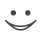# This flashcard is just one of a free flashcard set. See all flashcards!

234
15.Which of the following defines an Ito process?
A.A process where the drift is non-constant and can be stochastic
B.A process where the coefficient of dz is non-constant and can be stochastic
C.A process where either the drift or the coefficient of dz or both are non-constant and can be stochastic
D.A process  where proportional changes follow a generalized Wiener process